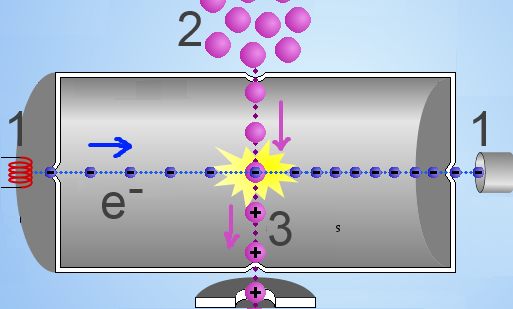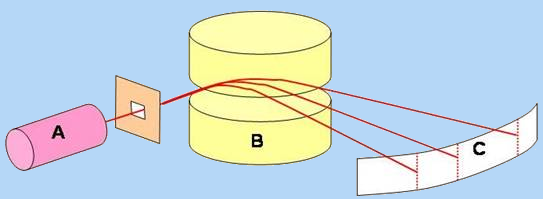Search:

# Mass spectroscopy

### Production of positive ionsA high voltage (1) throws electrons in a chamber where they collide violently with the molecules of a substance to be analyzed (2). Electrons are thus withdrawn from the body of the molecule which is transformed into a positive ion, said "molecular ion" (3), : $AB$ $+$ $e^-$ $\rightarrow$ $AB^+$ $+$ $2e^-$ The molecular ion is often unstable and breaks down into "molecular fragments" (3), some of which are positively charged: $AB^+$ $\rightarrow$ $A^+$ $+$ $B$ or $AB^+$ $\rightarrow$ $A$ $+$ $B^+$ The positive ions thus formed are accelerated to be analyzed subsequently.

### Analysis of the positive ionsA magnetic field B is used to separate the positive ions produced by A. Those whose ratio $\frac{mass}{charge}$ is the largest will be less deflected. Analyzer C is used to draw the mass spectrum :

### The mass spectrumThe molecular ion is obviously the heaviest, its charge equals 1, so it is the less deviated. Mass spectrometry is used to determine its molar mass (molecular peak), and therefore also that of the substance to be analysed. The deviation of the molecular fragments permits to determine their molar mass and guess what that fragments may be. The base peak corresponds to the most stable fragment (often stabilized by inductive or mesomeric effect). The abundance relative to the base peak allows classifying fragments according to their stability.